• http://blog.csdn.net/xiaofengsheng/article/details/6023368 ...  1. 一阶差分： ...2. 二阶偏导数的推导和近似：   3. 上式以点（i+1，j）为中心，用i代换i+1可得以（i,
http://blog.csdn.net/xiaofengsheng/article/details/6023368
http://www.cnblogs.com/german-iris/p/4840647.html

1. 一阶差分：2. 二阶偏导数的推导和近似：3. 上式以点（i+1，j）为中心，用i代换i+1可得以（i,j）为中心的二阶偏导数则有：4. 同理：5. 进而可推导：6. 这样我们就可以很好的运用其他的一阶偏导的定义，如SIFT特征OpenCV实现版本中的一阶以及二阶偏导：

[cpp]
view plain
copy

/*  Computes the partial derivatives in x, y, and scale of a pixel in the DoG  scale space pyramid.    @param dog_pyr DoG scale space pyramid  @param octv pixel's octave in dog_pyr  @param intvl pixel's interval in octv  @param r pixel's image row  @param c pixel's image col    @return Returns the vector of partial derivatives for pixel I      { dI/dx, dI/dy, dI/ds }^T as a CvMat*  */   static CvMat* deriv_3D( IplImage*** dog_pyr, int octv, int intvl, int r, int c )   {       CvMat* dI;       double dx, dy, ds;          dx = ( pixval32f( dog_pyr[octv][intvl], r, c+1 ) -           pixval32f( dog_pyr[octv][intvl], r, c-1 ) ) / 2.0;       dy = ( pixval32f( dog_pyr[octv][intvl], r+1, c ) -           pixval32f( dog_pyr[octv][intvl], r-1, c ) ) / 2.0;       ds = ( pixval32f( dog_pyr[octv][intvl+1], r, c ) -           pixval32f( dog_pyr[octv][intvl-1], r, c ) ) / 2.0;          dI = cvCreateMat( 3, 1, CV_64FC1 );       cvmSet( dI, 0, 0, dx );       cvmSet( dI, 1, 0, dy );       cvmSet( dI, 2, 0, ds );          return dI;   }            /*  Computes the 3D Hessian matrix for a pixel in the DoG scale space pyramid.    @param dog_pyr DoG scale space pyramid  @param octv pixel's octave in dog_pyr  @param intvl pixel's interval in octv  @param r pixel's image row  @param c pixel's image col    @return Returns the Hessian matrix (below) for pixel I as a CvMat*        / Ixx  Ixy  Ixs / <BR>      | Ixy  Iyy  Iys | <BR>      / Ixs  Iys  Iss /  */   static CvMat* hessian_3D( IplImage*** dog_pyr, int octv, int intvl, int r, int c )   {       CvMat* H;       double v, dxx, dyy, dss, dxy, dxs, dys;          v = pixval32f( dog_pyr[octv][intvl], r, c );       dxx = ( pixval32f( dog_pyr[octv][intvl], r, c+1 ) +                pixval32f( dog_pyr[octv][intvl], r, c-1 ) - 2 * v );       dyy = ( pixval32f( dog_pyr[octv][intvl], r+1, c ) +               pixval32f( dog_pyr[octv][intvl], r-1, c ) - 2 * v );       dss = ( pixval32f( dog_pyr[octv][intvl+1], r, c ) +               pixval32f( dog_pyr[octv][intvl-1], r, c ) - 2 * v );       dxy = ( pixval32f( dog_pyr[octv][intvl], r+1, c+1 ) -               pixval32f( dog_pyr[octv][intvl], r+1, c-1 ) -               pixval32f( dog_pyr[octv][intvl], r-1, c+1 ) +               pixval32f( dog_pyr[octv][intvl], r-1, c-1 ) ) / 4.0;       dxs = ( pixval32f( dog_pyr[octv][intvl+1], r, c+1 ) -               pixval32f( dog_pyr[octv][intvl+1], r, c-1 ) -               pixval32f( dog_pyr[octv][intvl-1], r, c+1 ) +               pixval32f( dog_pyr[octv][intvl-1], r, c-1 ) ) / 4.0;       dys = ( pixval32f( dog_pyr[octv][intvl+1], r+1, c ) -               pixval32f( dog_pyr[octv][intvl+1], r-1, c ) -               pixval32f( dog_pyr[octv][intvl-1], r+1, c ) +               pixval32f( dog_pyr[octv][intvl-1], r-1, c ) ) / 4.0;          H = cvCreateMat( 3, 3, CV_64FC1 );       cvmSet( H, 0, 0, dxx );       cvmSet( H, 0, 1, dxy );       cvmSet( H, 0, 2, dxs );       cvmSet( H, 1, 0, dxy );       cvmSet( H, 1, 1, dyy );       cvmSet( H, 1, 2, dys );       cvmSet( H, 2, 0, dxs );       cvmSet( H, 2, 1, dys );       cvmSet( H, 2, 2, dss );          return H;   }

参考：
（1）http://hi.baidu.com/shareshow/blog/item/34abdf544725cf54d109069b.html
（2）SIFT的OpenCV实现

OpenCV-跟我一起学数字图像处理之拉普拉斯算子

Laplace算子和Sobel算子一样，属于空间锐化滤波操作。起本质与前面的Spatial Filter操作大同小异，下面就通过Laplace算子来介绍一下空间锐化滤波，并对OpenCV中提供的Laplacian函数进行一些说明。
数学原理

离散函数导数

离散函数的导数退化成了差分，一维一阶差分公式和二阶差分公式分别为，Laplace算子的差分形式

分别对Laplace算子x,y两个方向的二阶导数进行差分就得到了离散函数的Laplace算子。
在一个二维函数f(x,y)中，x,y两个方向的二阶差分分别为，所以Laplace算子的差分形式为，0 1 0 1 -4 1 0 1 0

1 1 1 1 -8 1 1 1 1

注：

0 -1 0 -1 4 -1 0 -1 0

-1 -1 -1 -1 8 -1 -1 -1 -1

其原因是在定义二阶导数的时候采用了相反的定义，这个无关紧要，但是要注意，当用Laplace算子滤波后的图像与原图叠加时，混合操作是加还是减因上述的定义而异。

图像的Laplace操作

拉普拉斯操作结果与原图的混合

因为Laplace算子是二阶导数操作，其在强调图像素中灰度不连续的部分的同时也不在强调灰度值连续的部分。这样会产生一个具有很明显的灰度边界，但是没有足够特征的黑色背景。背景特征可以通过原图像与Laplace算子操作后的图像混合恢复。用公式，基于OpenCV的Laplace算子的计算
OpenCV中Laplacian函数可以实现对图像的Laplace操作，具体用法如下，

Laplacian( src_gray, dst, ddepth, kernel_size, scale, delta, BORDER_DEFAULT );

参数意义为，
基于OpenCV的Laplace算子仿真代码段如下，//load the Original Image and get some informations
namedWindow("OriginalImage");
imshow("OriginalImage",src);
CV_Assert(src.depth() == CV_8U);

//OpenCV solution - Laplacian
Mat dst,abs_dst_laplace;
Laplacian(src,dst,CV_16S,3);
convertScaleAbs(dst,abs_dst_laplace);

//show the result
namedWindow("result_laplacian");
imshow("result_laplacian",abs_dst_laplace);其中convertScaleAbs函数功能是将CV_16S型的输出图像转变成CV_8U型的图像。
仿真结果：
原图：Laplace操作结果：Laplace算子滤波仿真

根据数学原理中介绍的算法，编写相应代码，进行相关仿真。其中对Laplace操作结果进行了图像拉伸显示，因为Laplace操作结果的像素值范围可能落在了[0,255]之外，而计算机在显示的时候将赋值全部置为0，大于255的像素全部显示成255。
代码段如下，//get some informations of original image
int nr = src.rows;
int nc = src.cols;
int n = nr*nc;
int arr = {0};

//scan the whole pixels of original image
//and do Laplacian Operation
int* table_lap = new int[n];
int* table_orig = new int[n];
int l;
for (int i=0;i<n;i++)
{
table_lap[i] = 0;
table_orig[i] = 0;
}
for (int i=1;i<nr-1;i++)
{
const uchar* previous = src.ptr<uchar>(i-1);
const uchar* current = src.ptr<uchar>(i);
const uchar* next = src.ptr<uchar>(i+1);
for (int j=1;j<nc-1;j++)
{
for (int k=0;k<3;k++)
{
arr[k] = previous[j+k-1];
arr[k+3] = current[j+k-1];
arr[k+6] = next[j+k-1];
}
l = nc*i+j;        //calculate the location in the table of current pixel
table_orig[l] = arr;
}
}

//pixels scale
uchar* La_scaled = new uchar[n];
table_scale(table_lap,La_scaled,n);

Mat LaResult_own;
LaResult_own.create(src.size(),src.type());
uchar* p = NULL;
for (int i=0;i<nr;i++)
{
p = LaResult_own.ptr<uchar>(i);
for (int j=0;j<nc;j++)
{
l = nc*i+j;
p[j] = La_scaled[l];
}
}

//show results
namedWindow("LaResult_own");
imshow("LaResult_own",LaResult_own);//**********************//
//**********************//
{
int tmp = {-1,-1,-1,-1,8,-1,-1,-1,-1};
for (int i=0;i<9;i++)
{
table[l] = table[l] + tmp[i]*arr[i];
}
}tabel_scale函数就是我写的图像拉伸函数，将Laplace操作结果拉伸到[0,255]，具体函数段如下，//*****************************//
//scale the pixels to [0 255]
//*****************************//
void table_scale(int* table,uchar* result,int n)
{
int min = table;
int max = table;
for (int i=0;i<n;i++)
{
if(min>table[i])
{
min = table[i];
}
if(max<table[i])
{
max = table[i];
}
}
for (int i=0;i<n;i++)
{
result[i] = (uchar)(255*(table[i]-min)/(max-min));
}
}仿真结果，拉伸后Laplace算子的操作结果以灰色为主色调的显示结果就是Laplace算子操作拉伸后显示的一大特点。

Laplace滤波图像与原图像的混合//blending with the original image using Eq g(x,y)=f(x,y)+c*Lap(x,y)
int* table_blend = new int[n];
for(int i=0;i<n;i++)
{
table_blend[i] = table_orig[i] - table_lap[i];
if(table_blend[i]<0)
{
table_blend[i] = 0;
}
else if (table_blend[i]>255)
{
table_blend[i] = 255;
}
}

Mat Blresult;
Blresult.create(src.size(),src.type());
for (int i=0;i<nr;i++)
{
p = Blresult.ptr<uchar>(i);
for(int j=0;j<nc;j++)
{
l = nc*i+j;
p[j] = table_blend[l];
}
}

//show blending result
namedWindow("blending result_laplacian");
imshow("blending result_laplacian",Blresult);仿真结果：最后给出冈萨雷斯在介绍Laplacian时所给素材的仿真结果展开全文图像处理 opencv 卷积
• ## 高数——偏导数

万次阅读 多人点赞 2019-10-21 11:01:51
偏导数 在一元函数中，导数就是函数的变化率。对于二元函数研究它的“变化率”，由于自变量多了一个，情况就要复杂的多。 在 xOy 平面内，当动点由 P(x0,y0) 沿不同方向变化时，函数 f(x,y) 的变化快慢一般说来是...
偏导数
在一元函数中，导数就是函数的变化率。对于二元函数研究它的“变化率”，由于自变量多了一个，情况就要复杂的多。
在 xOy 平面内，当动点由 P(x0,y0) 沿不同方向变化时，函数 f(x,y) 的变化快慢一般说来是不同的，因此就需要研究 f(x,y) 在 (x0,y0) 点处沿不同方向的变化率。
在这里我们只学习函数 f(x,y) 沿着平行于 x 轴和平行于 y 轴两个特殊方位变动时， f(x,y) 的变化率。
偏导数的表示符号为:∂。
偏导数反映的是函数沿坐标轴正方向的变化率。
x方向的偏导
设有二元函数 z=f(x,y) ，点(x0,y0)是其定义域D 内一点。把 y 固定在 y0而让 x 在 x0有增量 △x ，相应地函数 z=f(x,y) 有增量（称为对 x 的偏增量）△z=f(x0+△x,y0)-f(x0,y0)。
偏导数如果 △z 与 △x 之比当 △x→0 时的极限存在，那么此极限值称为函数 z=f(x,y) 在 (x0,y0)处对 x 的偏导数，记作 f’x(x0,y0)或。函数 z=f(x,y) 在(x0,y0)处对 x 的偏导数，实际上就是把 y 固定在 y0看成常数后，一元函数z=f(x,y0)在 x0处的导数。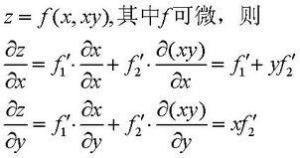y方向的偏导
同样，把 x 固定在 x0，让 y 有增量 △y ，如果极限存在那么此极限称为函数 z=(x,y) 在 (x0,y0)处对 y 的偏导数。记作f’y(x0,y0)。
相关求法
当函数 z=f(x,y) 在 (x0,y0)的两个偏导数 f’x(x0,y0) 与 f’y(x0,y0)都存在时，我们称 f(x,y) 在 (x0,y0)处可导。如果函数 f(x,y) 在域 D 的每一点均可导，那么称函数 f(x,y) 在域 D 可导。
此时，对应于域 D 的每一点 (x,y) ，必有一个对 x (对 y )的偏导数，因而在域 D 确定了一个新的二元函数，称为 f(x,y) 对 x (对 y )的偏导函数。简称偏导数。
按偏导数的定义，将多元函数关于一个自变量求偏导数时，就将其余的自变量看成常数，此时他的求导方法与一元函数导数的求法是一样的。
几何意义
表示固定面上一点的切线斜率。
偏导数 f’x(x0,y0) 表示固定面上一点对 x 轴的切线斜率；偏导数 f’y(x0,y0) 表示固定面上一点对 y 轴的切线斜率。
高阶偏导数：如果二元函数 z=f(x,y) 的偏导数 f’x(x,y) 与 f’y(x,y) 仍然可导，那么这两个偏导函数的偏导数称为 z=f(x,y) 的二阶偏导数。二元函数的二阶偏导数有四个：f"xx，f"xy，f"yx，f"yy。 注意：
f"xy与f"yx的区别在于：前者是先对 x 求偏导，然后将所得的偏导函数再对 y 求偏导；后者是先对 y 求偏导再对 x 求偏导。当 f"xy 与 f"yx 都连续时，求导的结果与先后次序无关。
假设ƒ是一个多元函数。例如：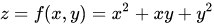因为曲面上的每一点都有无穷多条切线，描述这种函数的导数相当困难。偏导数就是选择其中一条切线，并求出它的斜率。通常，最感兴趣的是垂直于y轴（平行于xOz平面）的切线，以及垂直于x轴（平行于yOz平面）的切线。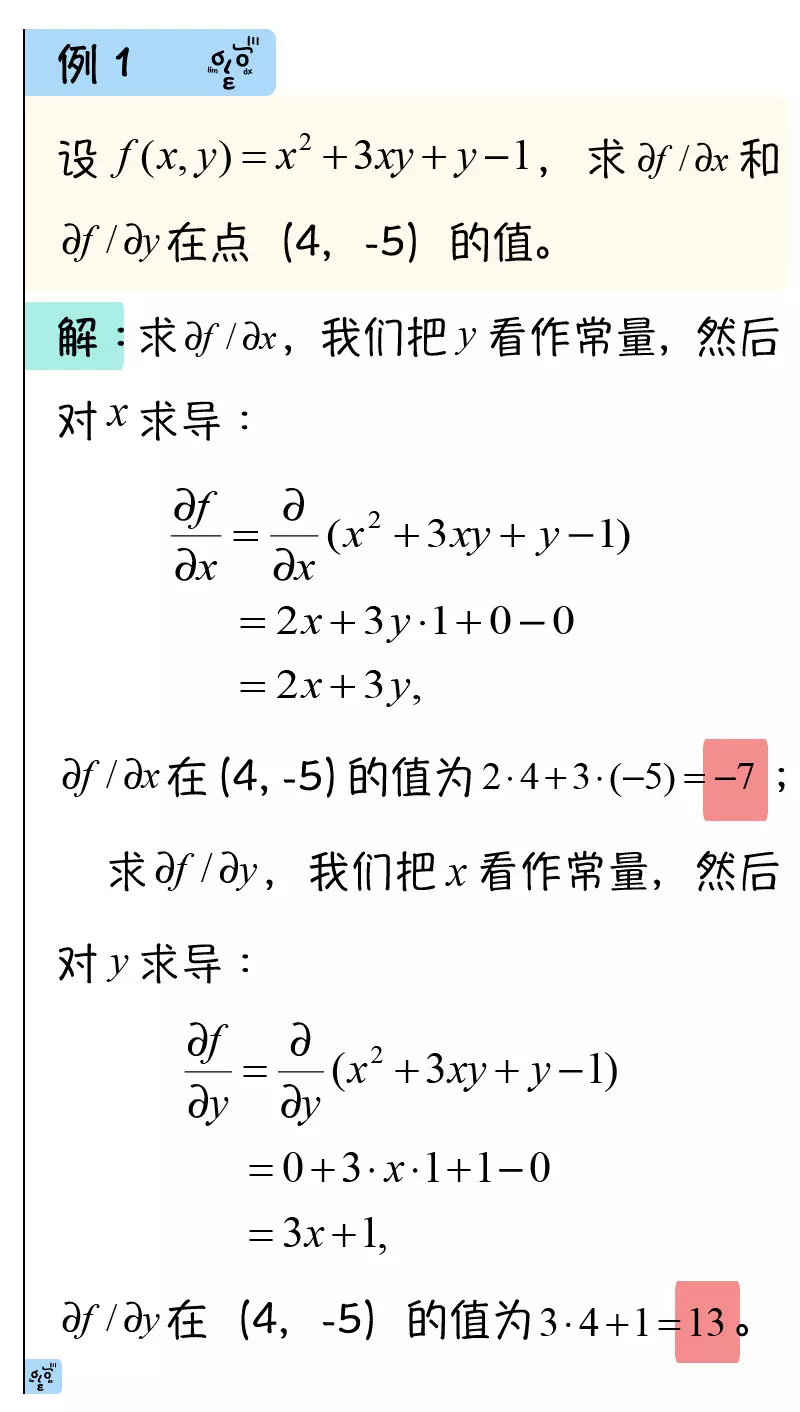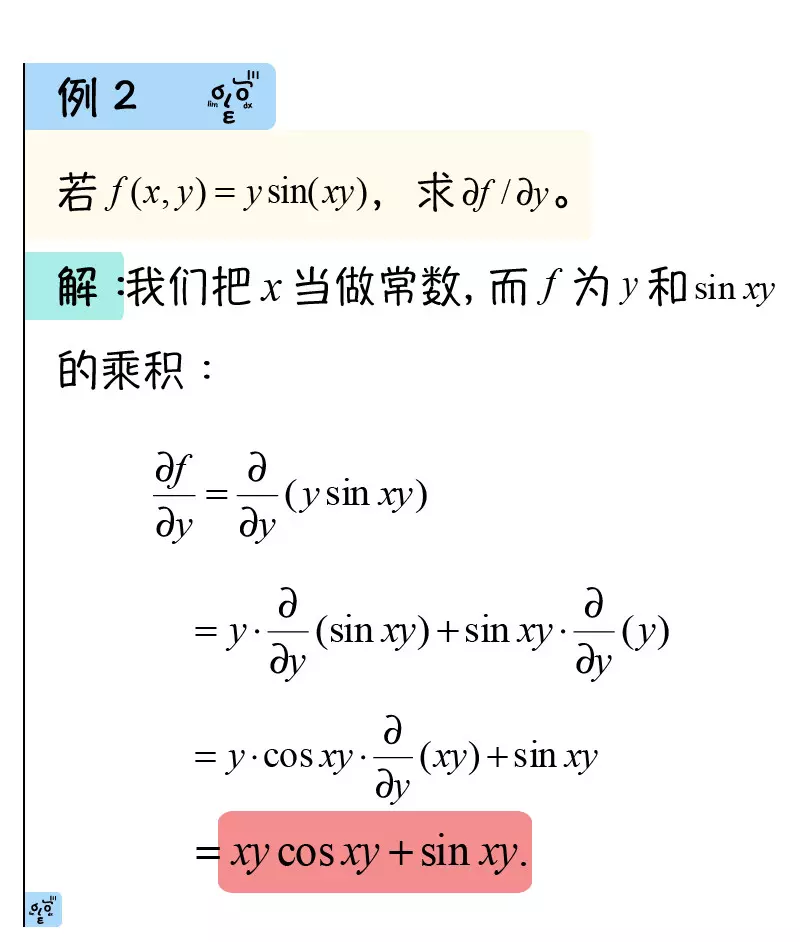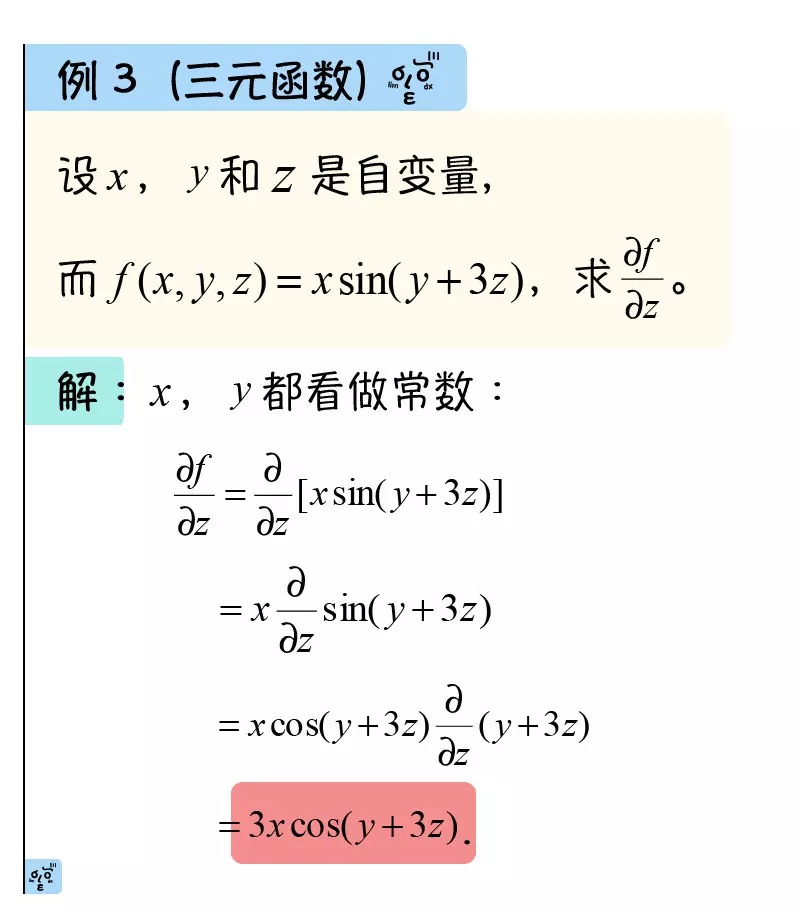本文转载自：https://www.jianshu.com/p/235495b42e59
展开全文• 学习到机器学习线性回归和逻辑回归时遇到了梯度下降算法，然后顺着扯出了一堆高数的相关概念理论：导数、偏导数、全微分、方向导数、梯度，重新回顾它们之间的一些关系，从网上和教材中摘录相关知识点。 通过函数...
学习到机器学习线性回归和逻辑回归时遇到了梯度下降算法，然后顺着扯出了一堆高数的相关概念理论：导数、偏导数、全微分、方向导数、梯度，重新回顾它们之间的一些关系，从网上和教材中摘录相关知识点。
通过函数的极限定义出导数(以一元函数为例)函数f(x)在点x0可微的充分必要条件是函数f(x)在点x0处可导扩展到多元函数时，衍生出偏导数
导数
定义：设函数

y

=

f

(

x

)

y=f(x)

在点

x

0

x_0

的某个领域内有定义，如果

Δ

y

Δ

x

\frac{Δy}{Δx}

在当

Δ

x

Δx

->0时极限存在，则称函数

y

=

f

(

x

)

y=f(x)

在

x

0

x_0

处可导，这个极限是函数

y

=

f

(

x

)

y=f(x)

在

x

0

x_0

处的导数

f

′

(

x

0

)

=

lim

⁡

Δ

x

→

0

Δ

y

Δ

x

=

lim

⁡

Δ

x

→

0

f

(

x

0

+

Δ

x

)

−

f

(

x

0

)

Δ

x

f&#x27;(x_0)=\lim \limits_{Δx \to 0} \frac{Δy}{Δx}=\lim \limits_{Δx \to 0} \frac{f(x_0+Δx)-f(x_0)}{Δx}

根据导数的定义，从某种意义上说导数的本质是一种极限

导数与导函数的关系是局部与整体的关系，导数通常是指一点，导函数则是指一个区间上的

在直线运动场景中，若x表示时刻，y表示距离，函数f表示时间与距离的关系

y

=

f

(

x

)

y=f(x)

,那么导数的含义就是在

x

0

x_0

时刻的瞬时速度在直角坐标系中，

y

=

f

(

x

)

y=f(x)

表示一个曲线，导数的含义表示的是曲线在点

x

0

x_0

处的切线的斜率
微分
定义：设函数

y

=

f

(

x

)

y=f(x)

在某个领域内有定义，

x

0

x_0

及

x

0

+

Δ

x

x_0+Δx

在这区间内，如果增量

Δ

y

=

f

(

x

0

+

x

)

−

f

(

x

0

)

Δy=f(x_0+x)-f(x_0)

可表示为

Δ

y

=

A

Δ

x

+

o

(

Δ

x

)

Δy=AΔx+o(Δx)

其中A是不依赖

Δ

x

Δx

的常数，

o

(

Δ

x

)

o(Δx)

是指

Δ

x

Δx

趋于0时的高阶无穷小，那么称函数

y

=

f

(

x

)

y=f(x)

在点

x

0

x_0

是可微的，而

A

Δ

x

AΔx

叫做函数在点

x

0

x_0

相应于自变量增量

Δ

x

Δx

的微分，记作

d

y

\mathrm{d} y

，记作

d

y

=

A

Δ

x

\mathrm{d}y=AΔx

高阶无穷小的定义：如果

lim

⁡

β

α

=

0

\lim \limits \frac{\beta}{\alpha}=0

，就说

β

\beta

是比

α

\alpha

高阶的无穷小，记作

β

=

o

(

α

)

\beta=o(\alpha)

微分与导数的关系
上式

Δ

y

=

A

Δ

x

+

o

(

Δ

x

)

Δy=AΔx+o(Δx)

两边同时除以

Δ

x

Δx

得到

Δ

y

Δ

x

=

A

+

o

(

Δ

x

)

Δ

x

\frac{Δy}{Δx}=A+\frac{o(Δx)}{Δx}

当

Δ

x

→

0

Δx \to 0

时,上式左边就是导数的定义，而右边的

o

(

Δ

x

)

Δ

x

\frac{o(Δx)}{Δx}

因为是高阶无穷小，所以会趋向于0，得到以下等式

A

=

lim

⁡

Δ

x

→

0

Δ

y

Δ

x

=

f

′

(

x

0

)

A=\lim \limits_{Δx \to 0}\frac{Δy}{Δx}=f&#x27;(x_0)

因此，如果函数

f

(

x

)

f(x)

在点

x

0

x_0

可微，则

f

(

x

)

f(x)

在点

x

0

x_0

也一定可导，且

A

=

f

′

(

x

0

)

A=f&#x27;(x_0)

，反之，如果

f

(

x

)

f(x)

在点

x

0

x_0

可导，存在下式

lim

⁡

Δ

x

→

0

Δ

y

Δ

x

=

f

′

(

x

0

)

\lim \limits_{Δx \to 0}\frac{Δy}{Δx}=f&#x27;(x_0)

根据极限与无穷小的关系转化上式，当

Δ

x

→

0

Δx \to 0

时

Δ

y

Δ

x

=

f

′

(

x

0

)

+

α

\frac{Δy}{Δx}=f&#x27;(x_0)+\alpha

其中

lim

⁡

Δ

x

→

0

a

=

0

\lim \limits_{Δx \to 0}a=0

，即

lim

⁡

Δ

x

→

0

a

Δ

x

Δ

x

=

0

\lim \limits_{Δx \to 0}\frac{aΔx}{Δx}=0

,

a

Δ

x

=

o

(

Δ

x

)

aΔx=o(Δx)

，上式转化为下式(又回到了微分的定义)

Δ

y

=

f

′

(

x

0

)

Δ

x

+

o

(

Δ

x

)

Δy=f&#x27;(x_0)Δx+o(Δx)

因此，函数

f

(

x

)

f(x)

在点

x

0

x_0

可微的充分必要条件是函数

f

(

x

)

f(x)

在点

x

0

x_0

可导

d

y

=

f

′

(

x

0

)

Δ

x

\mathrm{d}y=f&#x27;(x_0)Δx

偏导数
一元函数的变化率是导数，多元函数的自变量有多个，当某个自变量x变化而其它自变量固定时，这时候对变化的自变量x进行求导，就称为多元函数对于x的偏导数。 定义：设函数

z

=

f

(

x

,

y

)

z=f(x,y)

在点

(

x

0

,

y

0

)

(x_0,y_0)

的某一领域内有定义，当

y

y

固定于

y

0

y_0

，而

x

x

在

x

0

x_0

处有增量

Δ

x

Δx

，相应的函数有增量

f

(

x

0

+

Δ

x

,

y

0

)

−

f

(

x

0

,

y

0

)

f(x_0+Δx,y_0)-f(x_0,y_0)

如果

lim

⁡

Δ

x

→

0

f

(

x

0

+

Δ

x

,

y

0

)

−

f

(

x

0

,

y

0

)

Δ

x

\lim \limits_{Δx \to 0}\frac{f(x_0+Δx,y_0)-f(x_0,y_0)}{Δx}

存在，则称该极限为

z

=

f

(

x

,

y

)

z=f(x,y)

在点

(

x

0

,

y

0

)

(x_0,y_0)

处对

x

x

的偏导数
偏导数的几何意义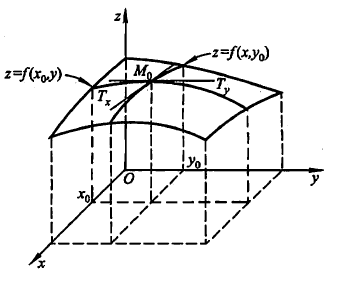偏导数

f

x

(

x

0

,

y

0

)

f_{x} (x_{0},y_{0} )

就是曲面被平面

y

=

y

0

y=y_{0}

所截得的曲线在点

M

0

M_{0}

处的切线

M

0

T

x

M_{0}T_{x}

对

x

x

轴的斜率偏导数

f

y

(

x

0

,

y

0

)

f_{y} (x_{0},y_{0} )

就是曲面被平面

x

=

x

0

x=x_{0}

所截得的曲线在点

M

0

M_{0}

处的切线

M

0

T

y

M_{0}T_{y}

对

y

y

轴的斜率
很多时候要考虑多元函数沿任意方向的变化率，那么就引出了方向导数
全微分
参考上文微分的定义，与一元函数的情形一样，希望用自变量增量

Δ

x

,

Δ

y

Δx,Δy

来线性函数来代替函数的全增量

Δ

z

Δz

，从而减化计算 定义：设函数

z

=

f

(

x

,

y

)

z=f(x,y)

在点

(

x

,

y

)

(x,y)

的某领域内有定义如果函数在点

(

x

,

y

)

(x,y)

的全增量

Δ

z

=

f

(

x

+

Δ

x

,

y

+

Δ

y

)

−

f

(

x

,

y

)

Δz=f(x+Δx,y+Δy)-f(x,y)

可心表示为

Δ

z

=

A

Δ

x

+

B

Δ

y

+

o

(

ρ

)

Δz=AΔx+BΔy+o(\rho)

其中

A

,

B

A,B

不依赖于

Δ

x

,

Δ

y

Δx,Δy

，

ρ

=

(

Δ

x

)

2

+

(

Δ

y

)

2

\rho=\sqrt{(Δx)^2+(Δy)^2}

，则称函数

z

=

f

(

x

,

y

)

z=f(x,y)

在点

(

x

,

y

)

(x,y)

处可微分，而

A

Δ

x

+

B

Δ

y

AΔx+BΔy

称为函数在点

(

x

,

y

)

(x,y)

的全微分

d

z

=

A

Δ

x

+

B

Δ

y

\mathrm{d}z=AΔx+BΔy

可微分与偏导数关系
基于上述全微分定义成立，存在某一点

p

′

(

x

+

Δ

x

,

y

+

Δ

y

)

p&#x27;(x+Δx,y+Δy)

对于式子

Δ

z

=

A

Δ

x

+

B

Δ

y

+

o

(

ρ

)

Δz=AΔx+BΔy+o(\rho)

也成立，当

Δ

y

=

0

Δy=0

时

f

(

Δ

x

+

x

,

y

)

−

f

(

x

,

y

)

=

A

Δ

X

+

o

(

∣

Δ

x

∣

)

f(Δx+x,y)-f(x,y)=AΔX+o(|Δx|)

两边除以

Δ

x

Δx

并且令

Δ

x

→

0

Δx \to 0

取极限

lim

⁡

Δ

x

→

0

f

(

x

+

Δ

x

,

y

)

−

f

(

x

,

y

)

Δ

x

=

A

\lim \limits_{Δx \to 0}\frac{f(x+Δx,y)-f(x,y)}{Δx}=A

这式子就是偏导数的定义形式啊，所以这说明了偏导数

f

x

(

x

,

y

)

f_x(x,y)

存在且等于

A

A

，同理也可证

f

y

(

x

,

y

)

=

B

f_y(x,y)=B

，由此推导出以下公式

d

z

=

f

x

(

x

,

y

)

Δ

x

+

f

y

(

x

,

y

)

Δ

y

\mathrm{d}z=f_x(x,y)Δx+f_y(x,y)Δy

各偏导数的存在只是全微分存在的必要条件而非充分条件，即由全微分可证各偏导数存在，反之则不行

如果函数的各个偏数在点

(

x

,

y

)

(x,y)

是连续的，则函数可微分

方向导数
定义导数、偏导数、方向导数都是说如果说某条件下极限存在，谨记导数的本质是极限及代表函数的变化率，偏导数反映的是函数沿坐标轴方向的变化率，有所限制，所以引入方向导数表示沿任意一方向的变化率 定义：设

l

l

是

x

O

y

xOy

平面以

P

0

(

x

0

,

y

0

)

P_0(x_0,y_0)

为始点的一条射线，

e

i

=

(

c

o

s

α

,

c

o

s

β

)

e_i=(cos\alpha,cos\beta)

是以射线同方向的单位向量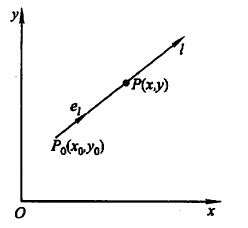射线

l

l

的参数方程为

{

x

=

x

0

+

t

c

o

s

α

，

t

≥

0

y

=

y

0

+

t

c

o

s

β

，

t

≥

0

\begin{cases}x=x_0+tcos\alpha ，t\geq0\\ y=y_0+tcos\beta，t\geq0 \end{cases}

如果函数增量

f

(

x

0

+

t

c

o

s

α

,

y

0

+

t

c

o

s

β

)

−

f

(

x

0

,

y

0

)

f(x_0+tcos\alpha,y_0+tcos\beta)-f(x_0,y_0)

与

P

P

到

P

0

P_0

的距离

∣

P

P

0

∣

=

t

|PP_0|=t

的比值，当点

P

P

沿着

l

l

趋于

P

0

(

即

t

→

0

+

)

P_0(即t \to 0^+)

时极限存在，则称此极限为函数在点

P

0

P_0

沿方向

l

l

的方向导数

∂

f

∂

l

∣

(

x

0

,

y

0

)

=

lim

⁡

t

→

0

+

f

(

x

0

+

t

c

o

s

α

,

y

0

+

t

c

o

s

β

)

−

f

(

x

0

,

y

0

)

t

\frac{\partial f}{\partial l}|_{(x_0,y_0)}=\lim \limits_{t \to 0^+}\frac{f(x_0+tcos\alpha,y_0+tcos\beta)-f(x_0,y_0)}{t}

方向导数与全微分的关系
由全微分的定义得到

f

(

x

0

+

Δ

x

,

y

0

+

Δ

y

)

−

f

(

x

0

,

y

0

)

=

f

x

(

x

0

,

y

0

)

Δ

x

+

f

y

(

x

0

,

y

0

)

Δ

y

+

o

(

(

Δ

x

)

2

+

(

Δ

y

)

2

)

f(x_0+Δx,y_0+Δy)-f(x_0,y_0)=f_x(x_0,y_0)Δx+f_y(x_0,y_0)Δy+o(\sqrt{(Δx)^2+(Δy)^2})

设点

(

x

0

+

Δ

x

,

y

0

+

Δ

y

)

(x_0+Δx,y_0+Δy)

在以

(

x

0

,

y

0

)

(x_0,y_0)

为起点的射线

l

(

c

o

s

α

,

c

o

s

β

是

l

的

方

向

余

弦

)

l(cos\alpha,cos\beta是l的方向余弦)

上，则有

Δ

x

=

t

c

o

s

α

Δx=tcos\alpha

,

Δ

y

=

t

c

o

s

β

Δy=tcos\beta

,

(

Δ

x

)

2

+

(

Δ

y

)

2

=

t

\sqrt{(Δx)^2+(Δy)^2}=t

，所以

lim

⁡

t

→

0

+

f

(

x

0

+

Δ

x

,

y

0

+

Δ

y

)

−

f

(

x

0

,

y

0

)

t

=

f

x

(

x

0

,

y

0

)

c

o

s

α

+

f

y

(

x

0

,

y

0

)

c

o

s

β

\lim \limits_{t \to 0^+}\frac{f(x_0+Δx,y_0+Δy)-f(x_0,y_0)}{t}=f_x(x_0,y_0)cos\alpha+f_y(x_0,y_0)cos\beta

上式左侧就是方向导数定义形式，极限存在即方向导数存在，且其值等于右式
由此得到定理，如果函数

f

(

x

,

y

)

f(x,y)

在点

P

0

(

x

0

,

y

0

)

P_0(x_0,y_0)

可微分，那么函数在该点沿任一方向

l

l

的方向导数存在

∂

f

∂

l

∣

(

x

0

,

y

0

)

=

f

x

(

x

0

,

y

0

)

c

o

s

α

+

f

y

(

x

0

,

y

0

)

c

o

s

β

\frac{\partial f}{\partial l}|_{(x_0,y_0)}=f_x(x_0,y_0)cos\alpha+f_y(x_0,y_0)cos\beta

梯度
在平面上确定某一点可能存在无数个方向导数，我们怎样找到其中一个方向导数来描述函数最大变化率？ 定义：在二元函数的情形， 设函数

f

(

x

,

y

)

f(x,y)

在平面区域D内具有一阶连续偏导数，对于每一点

P

0

(

x

0

,

y

0

)

∈

D

P_0(x_0,y_0)\in D

，都可以给出一个向量

f

x

(

x

0

,

y

0

)

i

+

f

y

(

x

0

,

y

0

)

j

或

用

坐

标

表

示

(

f

x

(

x

0

,

y

0

)

,

f

y

(

x

0

,

y

0

)

)

其中

i

,

j

i,j

为

x

,

y

x,y

轴的方向向量，上述微量称为函数

f

(

x

,

y

)

f(x,y)

在点

P

0

(

x

0

,

y

0

)

P_0(x_0,y_0)

的梯度记作

g

r

a

d

f

(

x

0

,

y

0

)

=

f

x

(

x

0

,

y

0

)

i

+

f

y

(

x

0

,

y

0

)

j

由定义看到，梯度的方向是确定的，如果点

P

P

的坐标确定，那么梯度也大小也确定
如果函数

f

(

x

,

y

)

f(x,y)

在点

P

0

(

x

0

,

y

0

)

P_0(x_0,y_0)

可微分，

e

l

=

(

c

o

s

α

,

c

o

s

β

)

e_l=(cos\alpha,cos\beta)

是方向

l

l

的方向向量(方向未确定)

∂

f

∂

l

∣

(

x

0

,

y

0

)

=

f

x

(

x

0

,

y

0

)

c

o

s

α

+

f

y

(

x

0

,

y

0

)

c

o

s

β

=

g

r

a

d

f

(

x

0

,

y

0

)

.

e

l

=

∣

g

r

a

d

f

(

x

0

,

y

0

)

∣

c

o

s

θ

其中

θ

\theta

为向量

g

r

a

d

f

(

x

0

,

y

0

)

与向量

e

l

e_l

的夹角，当

θ

=

0

\theta=0

时，即方向

e

l

e_l

与梯度

g

r

a

d

f

(

x

0

,

y

0

)

的方向时，函数

f

(

x

,

y

)

f(x,y)

增加最快，函数在这个方向的方向导数达到最大值，这个值就是梯度

g

r

a

d

f

(

x

0

,

y

0

)

的模，即

∂

f

∂

l

∣

(

x

0

,

y

0

)

=

∣

g

r

a

d

f

(

x

0

,

y

0

)

∣

所以可以用沿梯度方向的方向导数来描述是函数最大变化率，即梯度方向是函数变化率最大的方向，在梯度定义的时候就已经赋予了它这个特性。
展开全文高等数学 机器学习 梯度
• 偏导数全导数 偏导数 由于是二元函数，有两个因变量。偏导数表示分别对某一个导数求导，如偏x导数、偏y导数。 高阶偏导数偏导数继续求导。以二元函数的二阶偏导数为例，偏x导数有两个偏导数、偏y导数有两个偏导数...
偏导数全导数
偏导数
由于是二元函数，有两个因变量。偏导数表示分别对某一个导数求导，如偏x导数、偏y导数。
高阶偏导数
对偏导数继续求导。以二元函数的二阶偏导数为例，偏x导数有两个偏导数、偏y导数有两个偏导数。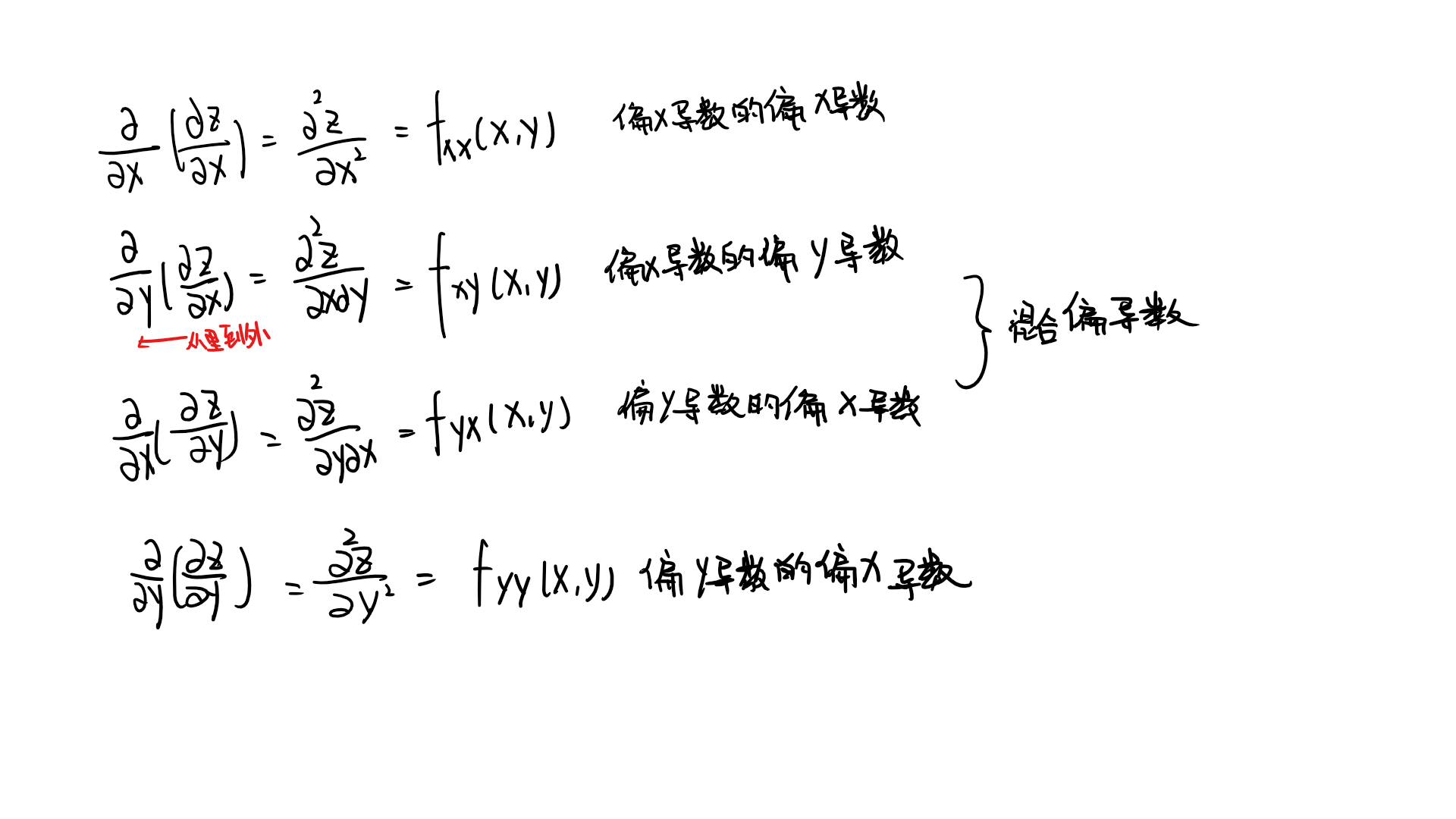定理：如果二元函数的两个二阶混合偏导数连续，那么他们两个相等。
全微分
与一元函数类似，由于有两个变量，x或y的增量称为偏增量，单单对x或y的微分称为偏微分。 若x，y同时增加，称为全增量。 全微分定义见下图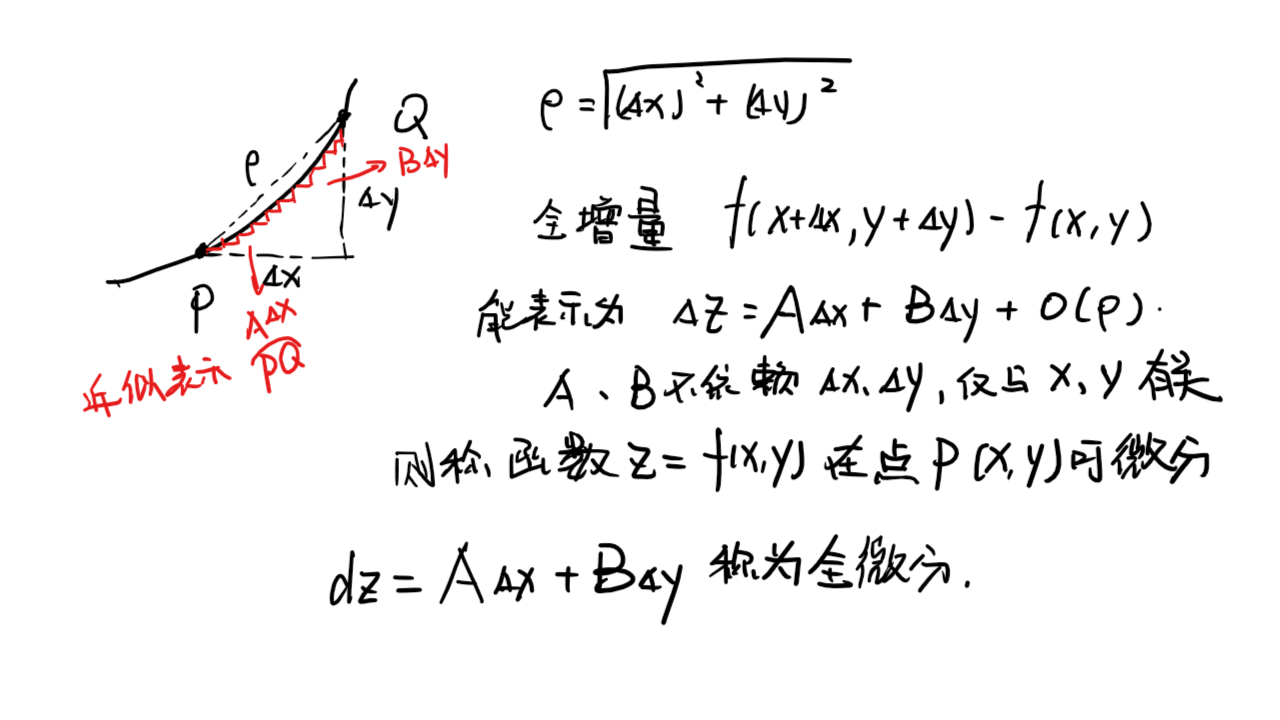定理
如果函数在该点可微分，那么其在该点的偏导数一定存在，且全微分中A、B分别等于偏x导数、偏y导数（叠加定理） （全微分存在，函数可微分，偏导数一定存在；偏导数存在，全微分不一定存在）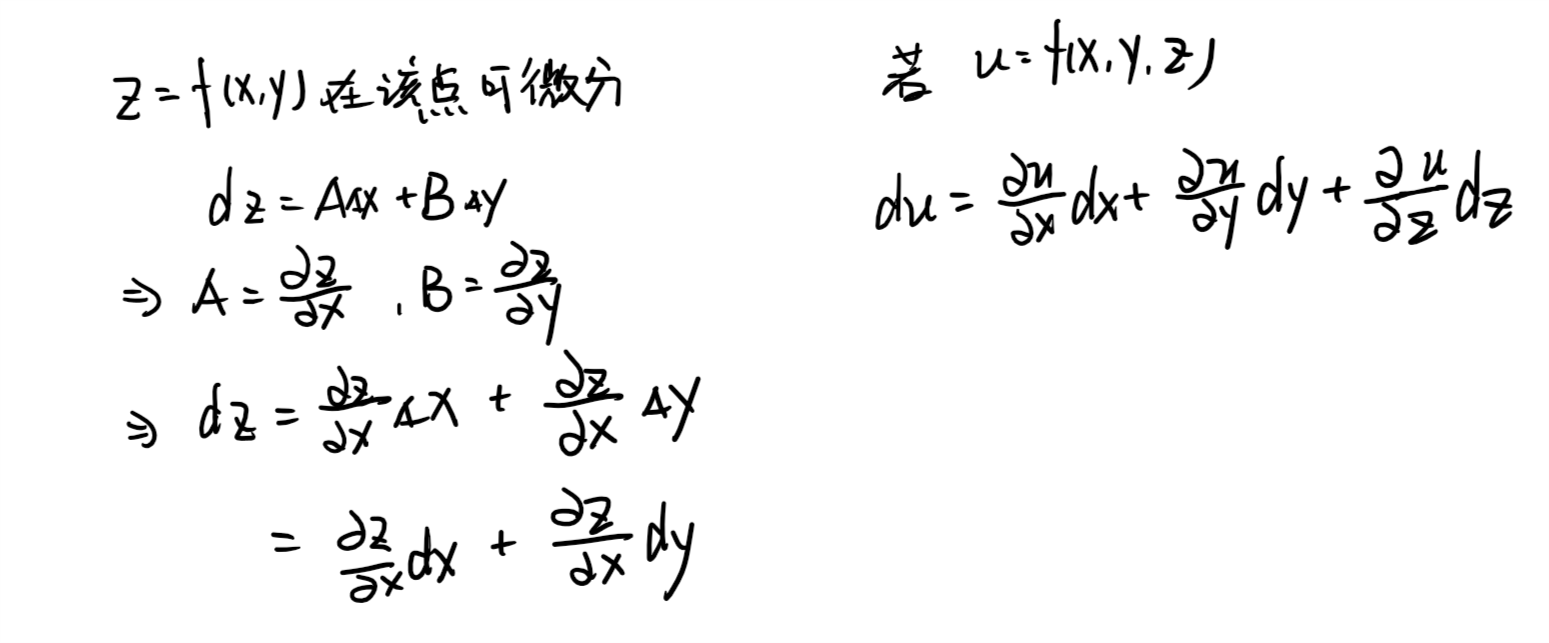如果函数在该点偏导数连续，那么函数在该点可微分
多元复合函数求导
一元函数与多元函数复合
先对多元函数微分，再把每个函数看成一元函数进行求导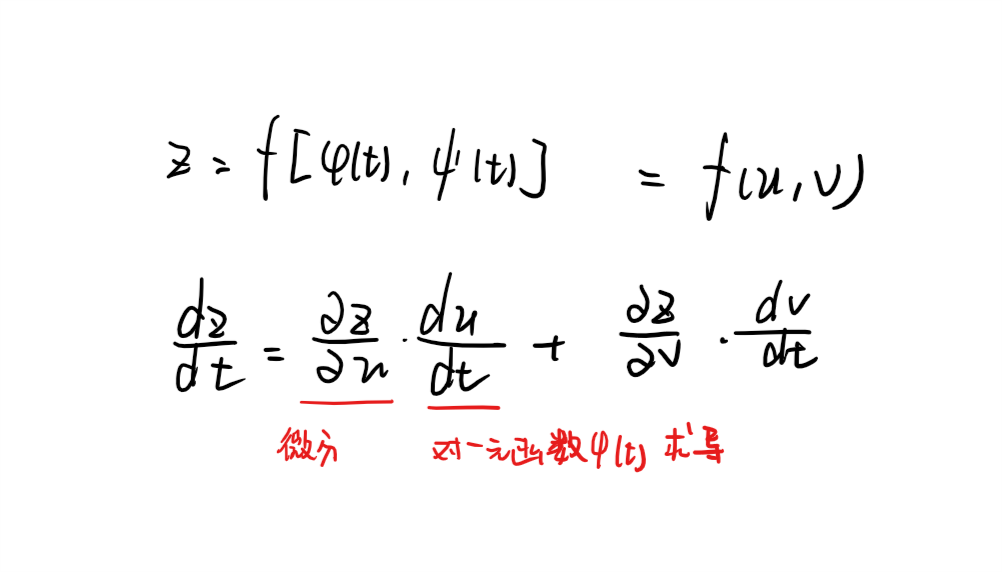多元函数与多元函数复合
如果对x求导，就先对所有函数微分，再把每个函数对x微分，最后相加。对y同理。其他情形
当多元函数与一元或者多元函数复合时，可能所导变量在某个函数中不存在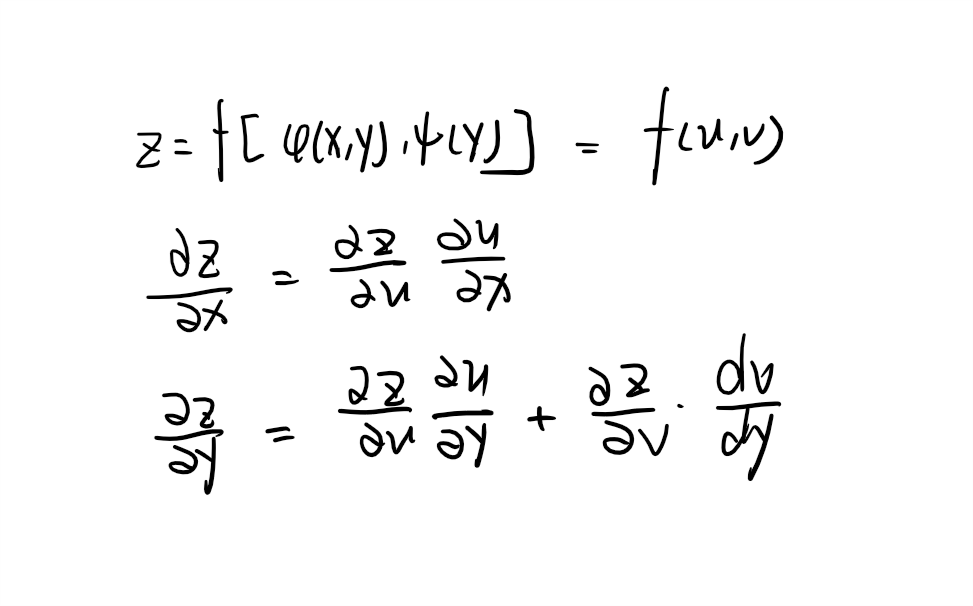不管那种情况，都有一下规律： 把最外层函数里的一个一个函数看过来，如果这个函数不存在所导变量，就不理他看下一个（微分后为0）。如果有，就先把最外层函数对其微分，如果里面这个函数是一元函数，就对变量求导；如果是多元，就对变量微分。
多元函数二阶求导
为方便起见，做出如下定义：有z=f(u,v)。f1’(u,v)=fu(u,v)——f对u求偏导;f2’=fv(u,v)——f对v求偏导;f12’’(u,v)=fuv(u,v)等等… 先求一阶偏导，再根据公式求二阶偏导数。需要注意的是此处求出来的是一阶偏导对变量的微分。由于一阶偏导内涵中间变量u、v，因此要再进行微分将一阶偏导对变量的微分变成二阶偏导。
隐函数求导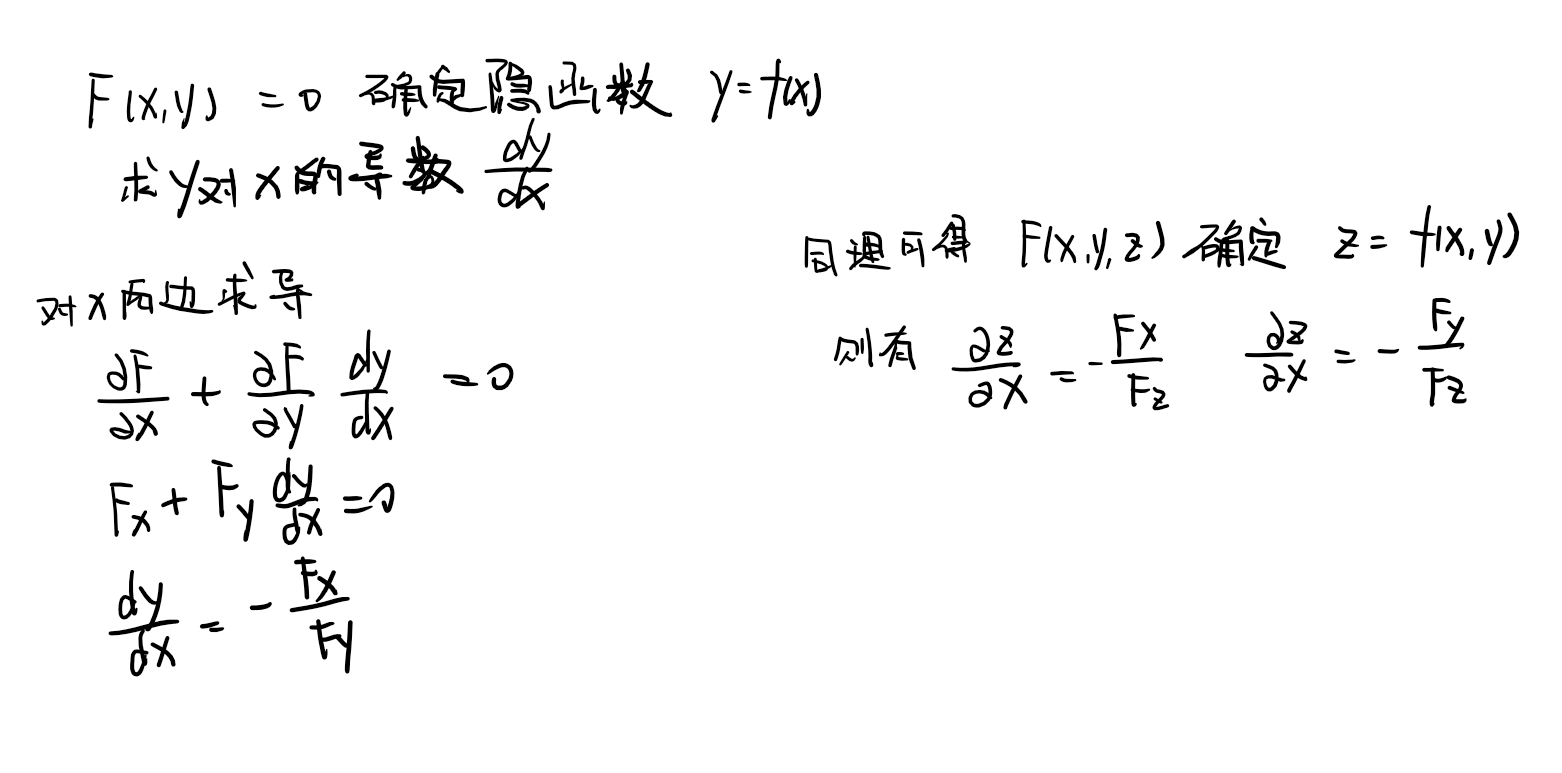方程组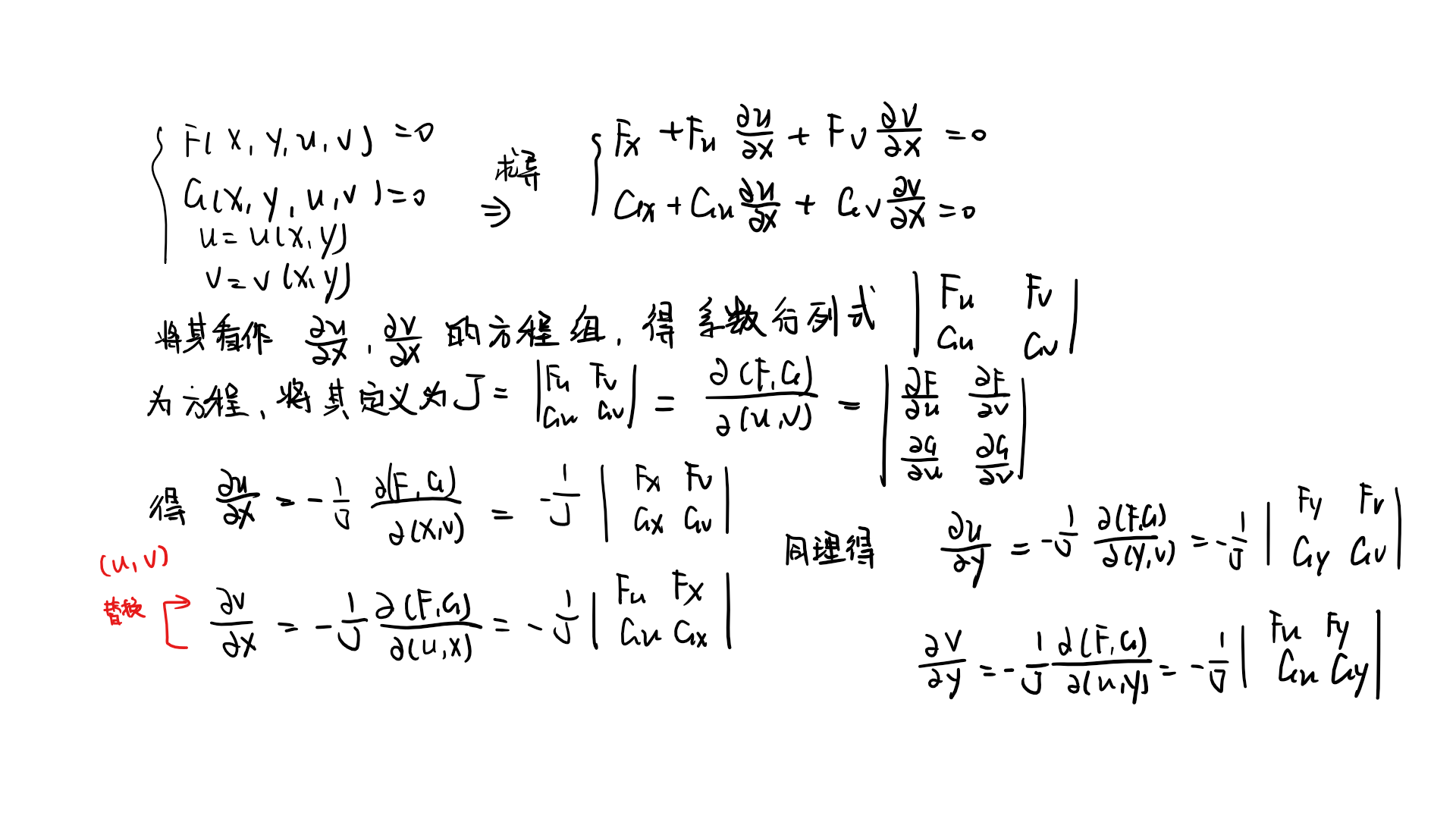在求解的时候可以把行列式右边的常数和所求的变量前的系数代换，利用行列式法则求解。 以下给出例题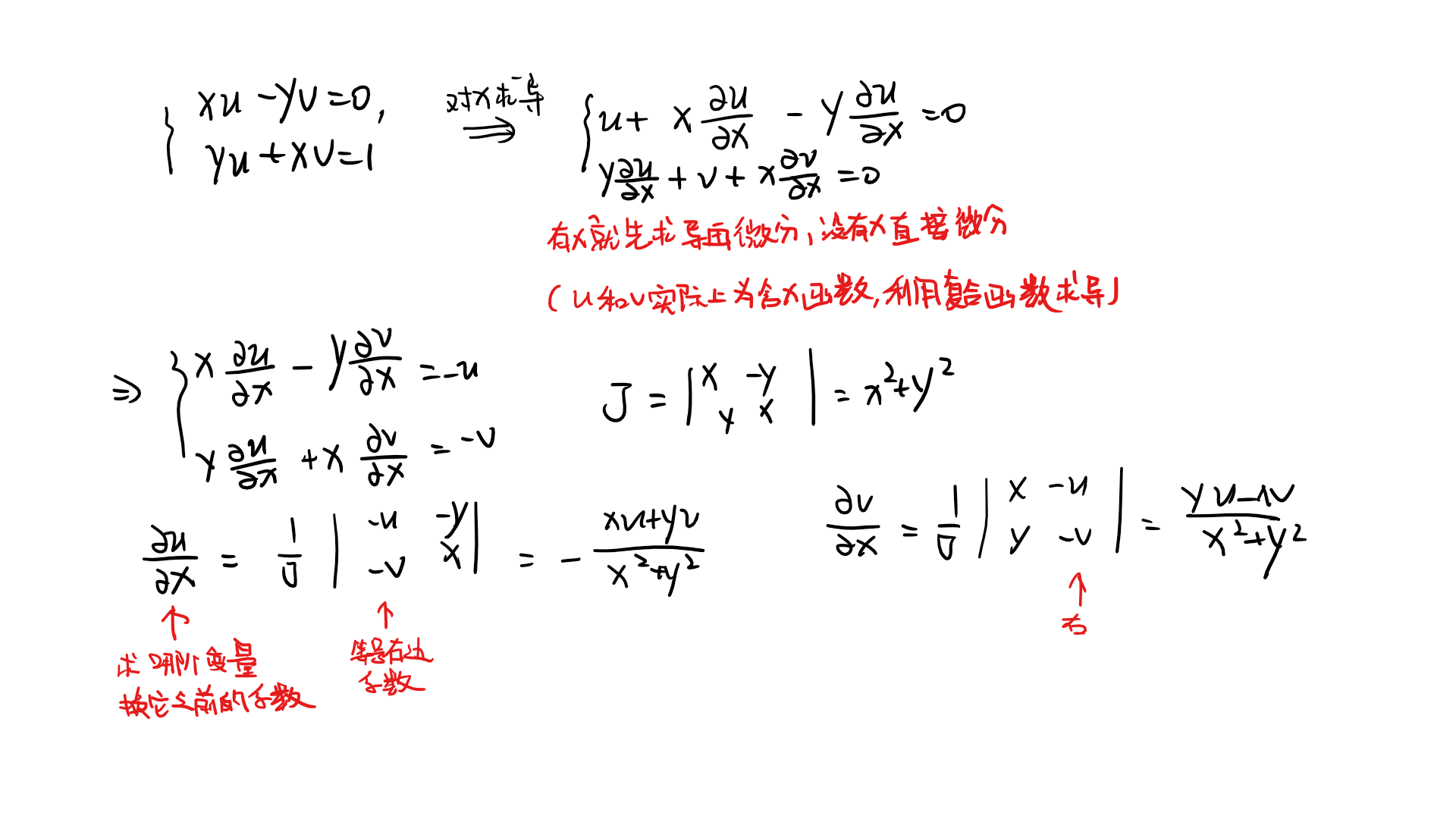展开全文高等数学 全导数 隐函数 多元函数
• 前一篇内容使用了大篇幅介绍一些基本概念，本篇则正式进入多元函数微分学的内容。 回顾 本篇内容为偏导数，在学习多元函数偏导数之前，先回顾一下一元函数的导数 ...二元函数偏导数定义 偏增量与全增量 ...
• 偏导数的概念 本质上就是求一元函数的导，只不过是把其他变量看作常数就行了。 在图像上显示可以想象下，例如z=f(x，y)这是一个三维 图形，然后对x求偏导其实就这一点所在的平行于zx平面的切面是投影到z，x上的...
• 参考博客...导数，是我们最早接触的一元函数中定义的，可以在 xy 平面直角坐标系中方便的观察。当 Δx→0时，P0处的导数就是因变量y在x0处的变化率，反映因变量...
• 1、导数 其实求导数是为了方便我们后来求极值，导数的几何意义指该函数曲线在这一点上的切线...如果当△x→ 时，△y/△x 之比的极限存在 则成为 y = f（x）在x₀处可，并且该极限值称为函数f（x）在点x₀处的...
• 偏导数 我们都知道导数是一元函数的变化率，衡量每个x位置处的瞬间变化率。 偏导数是针对多变量函数而言的，它通过将多变量函数退化成一元函数分别求各自的导数。以二元函数为例： Z = F（x，y） 求x的偏导数...
• 文章目录前言正文导数的定义导数的含义及常见函数导数导数的性质分数的求导导数和函数最小值的关系（重点）函数的增减表偏导数多变量函数偏导数的求解（重点）多变量函数的极值问题（重点）躲不掉的拉格朗日总结 ...神经网络 深度学习
• 美好的目标函数求未知数的偏导数是一个漂亮表达式，会让你惊叹到数学如此婀娜多娇。    如果有人能够求得未知变量的解析表达式，那该是多么幸福的事情，现实是残酷的。（我知道你想说伪逆是最小二乘的解析表达式...机器学习 深度学习 反向传播
• 1 连续的含义 通俗来说，用笔作画，不提笔画出来的曲线就是连续的： ...首先要对偏导数有所了解。多变量的函数f(x,y) 可以是三维空间中的曲面 https://blog.csdn.net/ccnt_2012/article/details/83310653 ...
• 偏导数： 假设 f(x,y)=x+y**2 偏导数就是在一个给定值（x0,y0）点初，先固定y的值，将y看成常数，对x求偏导相当于只有x一个自变量和求导数是一样的 再固定x ,将x看成常数，对y求偏导相当于只有y一个自变量和求...
• 　则根据偏导数定义得： 　同理可得： 2.MatMul（矩阵乘） 　矩阵乘的偏导与元素的顺序是相关的. 　设： 　那么： 　 　根据矩阵相容的原理： 　可见矩阵的偏导数是另一个矩阵的转置，但是在用...
• 2 ，二元函数偏导数 ：几何意义 偏导数 ： 对一个变量的导数 例子 ： 理解 ： 1 ，函数 z=f(x,y) 代表曲面 2 ，用 y=y0 截一下，得到一条曲线 3 ，在这条曲线上，对 x 求导，得到的依然是斜率 4 ，本质 ： 依然是...高等数学
• ## 偏导数的计算

千次阅读 2020-04-26 10:25:35
一阶偏导数 （1）定义式： 若极限不存在或不确定，则偏导数不存在。 注意： 另一个变量被当作常量看待，所以可将已知的数代入。 （2）求导公式： 其他变量均当做常数，按照一元函数求导数进行。 2. 高阶偏导数 二阶...
• 导数 导数反映的是函数y=f(x)在某一点处沿x轴正方向的变化率。...在多元函数中，偏导数指的是函数y(x1,x2,…,xn)沿某一坐标轴(x1,x2,…,xn)正方向的变化率。 比如z=x2+y2，在(1,2)处的在x方向上的偏...机器学习
• 学习到机器学习线性回归和逻辑回归时遇到了梯度下降算法，然后顺着扯出了一堆高数的相关概念理论：导数、偏导数、全微分、方向导数、梯度，重新回顾它们之间的一些关系，从网上和教材中摘录相关知识点。 这段是我...
• 基础数学4：导数、偏导数、方向导数、梯度、全微分回顾 （1）导数： ①导数的定义： 导数代表自变量的变换趋于无穷小时，函数值变化与自变量变化的比值，反映函数在某一点处沿x方向的变化率。 在一元函数中只...梯度
• 这里我们大致地复习一下偏导数，雅克比矩阵以及黑塞矩阵的定义和关系。 导数向量与雅克比矩阵（Jacobi matrix） 函数的某个因变量对某个自变量求的导数即为它们关于函数的偏导数。当因变量为一元的情况下，各个自...
• 导数： 定义： 设函数y= f（x）在点x₀的...如果当△x→ 时，△y/△x 之比的极限存在 则成为 y = f（x）在x₀处可，并且该极限值称为函数f（x）在点x₀处的导数。 f（x）= x² f`(x) = (x + △x)² - x²）/...
• 目录导数数值微分偏导数梯度（gradient） 导数 一个函数在某一点的导数描述了这个函数在这一点附近的变化率： 例如一元函数中，某一点的p导数，描述了该点切线的斜率： 数值微分 解析性求导 ：利用数学推导计算...
• 由于学习多变量微积分和电磁学时没有意识到数学基础的重要性，我对于矢量代数的理解一直不够透彻。...一个多变量函数的偏导数就是它在其它变量保持不变时，关于某一个变量的导数。它的记法有很多，两个变...数学 矢量代数
• 目录简化版教程知乎马同学教程 简化版教程 该教程提出导数的方向概念，导数可以认为是向量。...（转）导数、偏导数、方向导数、梯度、梯度下降 知乎马同学教程 微分和导数 全微分 全导数 梯度 ...
• 一篇经典博客： ... 1.导数定义： 导数代表了在自变量变化趋于无穷小的时候，函数值的变化...注意：在一元函数中，只有一个自变量变动，也就是说只存在一个方向的变化率，这也就是为什么一元函数没有偏导数的原因。 （de
• 包括全微分、偏导数、方向导数、梯度、全导数等内容。 初学这些知识的时候，学生会明显觉得这些概念不难掌握，而且定义及计算公式也很容易记住，但总觉得差那么点东西，说又不知道从何说起。反正笔者是这种感觉。...
•  它的核心观点是其中几个重要的公式用来实现Logistic回归梯度下降算法，但是在本文里面将使用导数流程图来计算梯度，必须承认用导数流程图来计算Logistic回归的梯度下降有点大材小用了，但是我认为以这种方式来讲解...
• 2.可微分定义判别法 3.全微分定理2中的有限增量公式(拉格朗日中值定理) 基础概念 二重极限： 求重极限： 初步判断看上下方幂：(最好还是代一下，记一般结论太随缘了。。) 证明判断是对的：取绝对值用夹逼...人工智能 算法 机器学习 编程语言
• 这里写自定义目录标题概述导数的概念偏导数的概念功能快捷键合理的创建标题，有助于目录的生成如何改变文本的样式插入链接与图片如何插入一段漂亮的代码片生成一个适合你的列表创建一个表格设定内容居中、居左、居右...方向导数 梯度
• 问题变了，思维方式就变了，之前的人的问题是什么什么是多少，他的问题是如何定义“什么什么”的问题。他定义的框架把微积分的严谨度向前迈了一大步，所以问什么样的问题最重要。微积分的现代体系就是他建的。 数学......